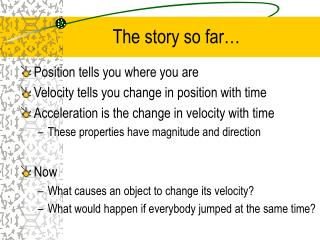DownloadDownload PresentationThe story so far…

The story so far…

Télécharger la présentationThe story so far…

- - - - - - - - - - - - - - - - - - - - - - - - - - - E N D - - - - - - - - - - - - - - - - - - - - - - - - - - -
Presentation Transcript

1. The story so far… • Position tells you where you are • Velocity tells you change in position with time • Acceleration is the change in velocity with time • These properties have magnitude and direction • Now • What causes an object to change its velocity? • What would happen if everybody jumped at the same time?

2. Newton’s First law The Law of Inertia

3. AristotleGreek Scientist of the 4th Century B.C.Motion is divided into two types • Natural Motion – Straight up or straight down • Objects seek out their natural resting place. • Violent Motion – Imposed motion • Result of a push or pull • The result of motion is from an external cause

4. To Sum Up Aristotle • The proper state of objects is at rest. • Unless an object is being pushed or pulled (violent motion), it is seeking its natural resting place. • Earth did not move, it was in its natural resting state, of course.

5. Copernicus The Earth moves!!!!!!

6. De Revolutionibus • The Earth and planets move around the sun. • The idea was very controversial and Copernicus was reluctant to publish his theory, in fear of being prosecuted. • On the day of his death, the first copy was delivered to him.

7. Galileo on Motion Galileo asked how things move, not why they moved. An object's velocity will not change all on its own. Pushes, or pulls, are necessary to change an object's velocity.

8. Galileo • Inertia is a property of matter. It is that property of matter which opposes changes in velocity. Simply stated, a common object will not change its velocity spontaneously. • Friction is the name given to the force that acts between materials that touch as they move past one another. • Argued that when friction is present a force is needed to keep an object moving.

9. Newton’s First Law

10. The Law of Inertia • Every object continues in a state of rest, or of motion in a straight line at constant speed, unless it is compelled to change that state by forces exerted upon it. • In simple terms, everything is lazy. Matter wants to keep doing what it’s doing until it is forced to change.

11. First law in Motion http://www.glenbrook.k12.il.us/gbssci/phys/mmedia/newtlaws/mb.html

12. MASS A Measure of Inertia

13. Mass – It’s Not Volume • Mass is a measure of inertia. • Volume is a measure of how much space an object takes up. (cc, liters, cubic meters) • Mass is measured in kilograms. • An object’s mass cannot always tell you about it’s volume.

14. Mass is not Weight • Weight is the force of gravity on an object. • When we say, “I’ve gained weight” or “I need to lose some weight”, we really mean mass. • In a gravity free environment, there is no weight. • Even though mass and weight are not the same, they are proportional.

15. SI • Mass is measured in kilograms. 1 kg is equal to 2.2 pounds • Weight is measured in Newtons 9.8 Newton’s are equal to 1 kg on earth • Weight is equal to gravity times mass w=mg

16. Equilibrium • When an object is at rest or moving at constant velocity there is no net force acting on it. • There may be forces acting on the object, but the total force is zero. • All the forces balance out • Use a freebody diagram to show the forces

17. Freebody Diagram of book on the table Fg = weight FN = normal force FN Fg